# Catenary

Catenary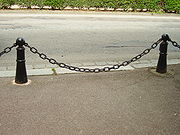A hanging chain forms a catenary.The silk on a spider's web forming multiple (approximate) catenaries.

In physics and geometry, the catenary is the curve that an idealised hanging chain or cable assumes when supported at its ends and acted on only by its own weight. The curve is the graph of the hyperbolic cosine function, and has a U-like shape, superficially similar in appearance to a parabola (though mathematically quite different). Its surface of revolution, the catenoid, is a minimal surface and is the shape assumed by a soap film bounded by two parallel circular rings.

## History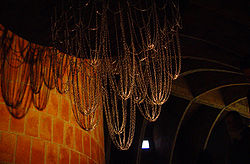Gaudi's catenary model at Casa Milà

The word catenary is derived from the Latin word catena, which means "chain". Thomas Jefferson is usually credited with the English word catenary. This occurs in a passage in a letter to Thomas Paine on the construction of an arch for a bridge.

I have lately received from Italy a treatise on the equilibrium of arches, by the Abbé Mascheroni. It appears to be a very scientifical work. I have not yet had time to engage in it; but I find that the conclusions of his demonstrations are, that every part of the catenary is in perfect equilibrium.

The curve is also called the "alysoid", "chainette", or, particularly in the material sciences, "funicular".

It is often stated that Galileo thought that the curve followed by a hanging chain is a parabola. A careful reading of his book Two new sciences shows this to be an oversimplification. Galileo states that a hanging cord is approximated by a parabola, correctly observing that this approximation improves as the curvature gets smaller and is almost exact when the elevation is less than 45°.  That the curve followed by a chain is not a parabola was proven by Joachim Jungius (1587–1657) and published posthumously in 1669.

The application of the catenary to the construction of arches is due to Robert Hooke, who discovered it in the context of the rebuilding of St Paul's Cathedral, possibly having seen Huygens' work on the catenary. (Some much older arches are also approximate catenaries.)

In 1671, Hooke announced to the Royal Society that he had solved the problem of the optimal shape of an arch, and in 1675 published an encrypted solution as a Latin anagram in an appendix to his Description of Helioscopes, where he wrote that he had found "a true mathematical and mechanical form of all manner of Arches for Building." He did not publish the solution of this anagram in his lifetime, but in 1705 his executor provided it as Ut pendet continuum flexile, sic stabit contiguum rigidum inversum, meaning "As hangs a flexible cable so, inverted, stand the touching pieces of an arch."

In 1691 Gottfried Leibniz, Christiaan Huygens, and Johann Bernoulli derived the equation in response to a challenge by Jakob Bernoulli. David Gregory wrote a treatise on the catenary in 1697.

Euler proved in 1744 that the catenary is the curve which, when rotated about the x-axis, gives the surface of minimum surface area (the catenoid) for the given bounding circles. Nicolas Fuss gave equations describing the equilibrium of a chain under any force in 1796.

## The inverted catenary archArch of Taq-i Kisra in Ctesiphon as seen today is roughly but not exactly a catenary.

Catenary arches are often used in the construction of kilns. In this construction technique, the shape of a hanging chain of the desired dimensions is transferred to a form which is then used as a guide for the placement of bricks or other building material.

The Gateway Arch in St. Louis, Missouri, United States is sometimes said to be an (inverted) catenary, but this is incorrect. It is close to a more general curve called a flattened catenary, with equation y=Acosh(Bx). (A catenary would have AB=1.) While a catenary is the ideal shape for a freestanding arch of constant thickness, the Gateway Arch is narrower near the top. According to the U.S. National Historic Landmark nomination for the arch, it is a "weighted catenary" instead. Its shape corresponds to the shape that a weighted chain, having lighter links in the middle, would form.

Inverted catenary arches
 Catenary arches under the roof of Gaudí's Casa Milà, Barcelona, Spain.
 The Sheffield Winter Garden is enclosed by a series of catenary arches.
 The Gateway Arch (looking East) is a flattened catenary.
 Catenary arch kiln under construction over temporary form

## Catenary bridges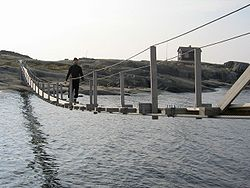Simple suspension bridges are essentially thickened cables, and follow a catenary curve.

In free-hanging chains the force exerted is uniform with respect to length of the chain and so the chain follows the catenary curve. The same is true of a simple suspension bridge or "catenary bridge," where the roadway follows the cable.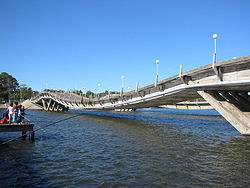Stressed ribbon bridges, like this one in Maldonado, Uruguay, also follow a catenary curve, with cables embedded in a rigid deck.

A stressed ribbon bridge is a more sophisticated structure with the same catenary shape.

However in a suspension bridge with a suspended roadway, the chains or cables support the weight of the bridge, and so do not hang freely. In most cases the roadway is flat, so when the weight of the cable is negligible compared with the weight being supported, the force exerted is uniform with respect to horizontal distance, and the result is a parabola, as discussed below (although the term "catenary" is often still used, in an informal sense). If the cable is heavy then the resulting curve is between a catenary and a parabola.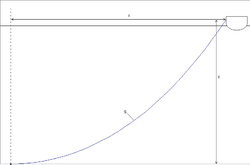A heavy anchor chain forms a catenary, with a low angle of pull on the anchor.

## Anchoring of marine objects

The catenary form given by gravity is taken advantage of in its presence in heavy anchor rodes. An anchor rode (or anchor line) usually consists of chain and/or cable. Anchor rodes are used by ships, oilrigs, docks, floating wind turbines, and other marine assets which must be anchored to the seabed.

When the rode is slack, the catenary curve presents a lower angle of pull on the anchor or mooring device than would be the case if it was nearly straight. This assists the performance of the anchor and raises the level of force it will resist before dragging. To maintain the catenary shape in the presence of wind, a heavy chain is needed, so that only larger ships in deeper water can rely on this effect – smaller boats must rely on the performance of the anchor itself.

## Mathematical description

### Equation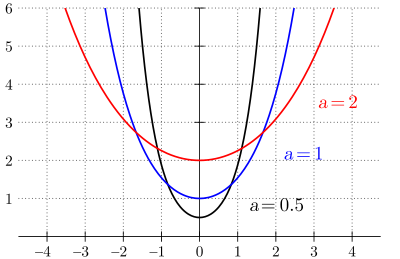Catenaries for different values of a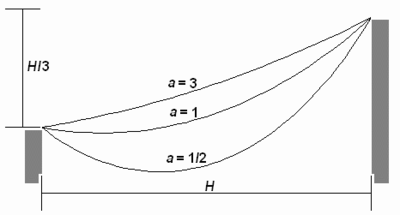Three different catenaries through the same two points, depending horizontal force$T_H$, being$a = \lambda H/T_H$ and λ mass per unit length.

The equation of a catenary in Cartesian coordinates has the form$y = a \, \cosh \left ({x \over a} \right ) = {a \over 2} \, \left (e^{x/a} + e^{-x/a} \right )$,

where cosh is the hyperbolic cosine function.

The Whewell equation for the catenary is$\tan \varphi = \frac{s}{a}$.

Differentiating gives$\frac{d\varphi}{ds} = \frac{\cos^2\varphi}{a}$

and eliminating φ gives the Cesàro equation$\kappa=\frac{a}{s^2+a^2}$.

The radius of curvature is then

ρ = asec 2φ

which is the length of the line normal to the curve between it and the x-axis.

### Other properties

All catenary curves are similar to each other. Changing the parameter a is equivalent to a uniform scaling of the curve.

A parabola rolled along a straight line traces out a catenary (see roulette) with its focus.

Square wheels can roll perfectly smoothly if the road has evenly spaced bumps in the shape of a series of inverted catenary curves. The wheels can be any regular polygon except a triangle, but the catenary must have parameters corresponding to the shape and dimensions of the wheels.

A charge in a uniform electric field moves along a catenary (which tends to a parabola if the charge velocity is much less than the speed of light c).

The surface of revolution with fixed radii at either end that has minimum surface area is a catenary revolved about the x-axis.

Over any horizontal interval, the ratio of the area under the caternary to its length equals a, independent of the interval selected. The catenary is the only plane curve other than a horizontal line with this property. Also, the geometric centroid of the area under a stretch of catenary is the midpoint of the perpendicular segment connecting the centroid of the curve itself and the x-axis.

## Analysis

### Model of chains and arches

The chain (or cord, cable, rope, string, etc.) is idealized by assuming that it is so thin that it can be regarded as a curve and that it is so flexible any force exerted by the chain itself is parallel to the chain. The analysis of the curve for an optimal arch is similar except that the forces of tension become forces of compression and everything is inverted. An underlying principle is that the chain may be considered a rigid body once it has attained equilibrium. Equations which define the shape of the curve and the tension of the chain at each point may be derived by a careful inspection of the various forces acting on a segment using the fact that these forces must be in balance if the chain is in static equilibrium.

Let the path followed by the chain is given parametrically by r = (x, y) = (x(s), y(s)) where s represents arc length and r is the position vector. This is the natural parameterization and has the property that$\frac{d\mathbf{r}}{ds}=\mathbf{u}$

where u is a unit tangent vector.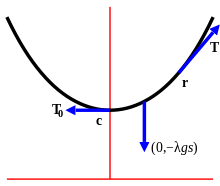Diagram of forces acting on a segment of a catenary from c to r. The forces are the tension T0 at c, the tension T at r, and the weight of the chain (0, −λgs). Since the chain is at rest the sum of these forces must be zero.

A differential equation for the curve may be derived as follows. Let c be the lowest point on the chain, called the vertex of the catenary,  and measure the parameter s from c. Assume r is to the right of c since the other case is implied by symmetry. The forces acting on section of the chain from c to r are the tension of the chain at c, the tension of the chain at r and the weight of the chain. The tension at c is tangent to the curve at c and is therefore horizontal, and it pulls the section to the left so it may be written (−T0, 0) where T0 is the magnitude of the force. The tension at r is parallel to the curve at r and pulls the section to the right, so it may be written Tu=(Tcos φ, Tsin φ), where T is the magnitude of the force and φ is the is the angle between the curve at r and the x-axis (see tangential angle). Finally, weight of the chain is represented by (0, −λgs) where λ is the mass per unit length and g is the acceleration of gravity.

The chain is in equilibrium so the sum of three forces is 0, therefore

Tcos φ = T0
Tsin φ = λgs

and dividing these$\frac{dy}{dx}=\tan \varphi = \frac{\lambda gs}{T_0}$

It is convenient to write$a = \frac{T_0}{\lambda g}$

which is the length of chain whose weight is equal in magnitude to the tension at c.  Then$\frac{dy}{dx}=\frac{s}{a}$

is an equation defining the curve.

It is immediate that the horizontal component of the tension, Tcos φ = λga is constant and the vertical component of the tension, Tsin φ = λgs is proportional to the length of chain between the r and the vertex.

### Derivation of equations for the curve

The differential equation given above can be solved to produce equations for the curve. 

From$\frac{dy}{dx} = \frac{s}{a},$$\frac{ds}{dx} = \sqrt{1+\left(\dfrac{dy}{dx}\right)^2} = \frac{\sqrt{a^2+s^2}}{a}.$

Then$\frac{dx}{ds} = \frac{1}{\frac{ds}{dx}} = \frac{a}{\sqrt{a^2+s^2}}$

and$\frac{dy}{ds} = \frac{\frac{dy}{dx}}{\frac{ds}{dx}} = \frac{s}{\sqrt{a^2+s^2}}$.

The second of these equations can be integrated to give$y = \sqrt{a^2+s^2} + \beta$

and by shifting the position of the x-axis, β can be taken to be 0. Then$y = \sqrt{a^2+s^2},\ y^2=a^2+s^2$

The x-axis thus chosen is called the directrix of the catenary.

It follows that the magnitude of the tension at a point T = λgy which is proportional to the distance between the point and the directrix.

The integral of expression for dx/ds can be found using standard techniques giving$x = a\ \operatorname{arcsinh}(s/a) + \alpha$.

and, again, by shifting the position of the y-axis, α can be taken to be 0. Then$x = a\ \operatorname{arcsinh}(s/a),\ s=a \sinh{x \over a}$.

The y-axis thus chosen passes though the vertex and is called the axis of the catenary.

These results can be used to eliminate s giving$y = a \cosh \frac{x}{a}.$

### Alternative derivation

The differential equation can be solved using a different approach.

From

s = atan φ

it follows$\frac{dx}{d\varphi} = \frac{dx}{ds}\frac{ds}{d\varphi}=\cos \varphi \cdot a \sec^2 \varphi= a \sec \varphi$$\frac{dy}{d\varphi} = \frac{dy}{ds}\frac{ds}{d\varphi}=\sin \varphi \cdot a \sec^2 \varphi= a \tan \varphi \sec \varphi.$

Integrating,

x = aln(sec φ + tan φ) + α
y = asec φ + β.

As before, the x and y-axes can be shifted so α and β can be taken to be 0. Then

sec φ + tan φ = ex / a
sec φ − tan φ = e x / a$y = a \sec \varphi = a \cosh \tfrac{x}{a}$$s = a \tan \varphi = a \sinh \tfrac{x}{a}.$

### Determining parameters

The equation of a catenary suspended at given points A and A′ and with given length l can be determined as follows: Relabel if necessary so that A is to the left of A′ and let h and k be the vertical and horizontal distances from A to A′. Translate the axes so that the origin is at the vertex of the catenary so the equation of the curve is$y = a \cosh \tfrac{x}{a}$

and let the coordinates of A and A′ be (bc) and (b′c′) respectively. The curve passes through these points, so$c = a \cosh \tfrac{b}{a},\,c' = a \cosh \tfrac{b'}{a},$$k = a (\cosh \tfrac{b}{a} - \cosh \tfrac{b'}{a}).$

The lengths of the curve from the vertex to A and from the vertex to A′ are$a \sinh \tfrac{b}{a},\, a \sinh \tfrac{b'}{a}$

respectively, so the length from A to A′ is$l = a (\sinh \tfrac{b}{a} - \sinh \tfrac{b'}{a}).$

Then$l^2-k^2=a^2(-2+2\cosh \tfrac{b}{a}\cosh \tfrac{b'}{a}-2\sinh \tfrac{b}{a} \sinh \tfrac{b'}{a})=a^2(-2+2\cosh \tfrac{b+b'}{a})=4a^2\sinh^2 \tfrac{h}{2a},$$\sqrt{l^2-k^2}=2a\sinh \tfrac{h}{2a}.$

This is a transcendental equation in a and must be solved numerically. However, it can be shown with the methods of calculus that there is at most one solution with a>0 and so there is at most one position of equilibrium. Furthermore, a solution exists only when$\sqrt{l^2-k^2}>h,$

in other words l is greater than the distance from A to A′. That is, a solution exists only when the length of the chain is longer than the distance between the two points.

## Generalizations with vertical force

### Nonuniform chains

If the density of the chain is variable then the analysis above can be adapted to produce equations for the curve given the density, or given the curve to find the density.

Let w denote the weight per unit length of the chain, then the weight of the chain has magnitude$\int w\ ds$

where the limits of integration are c and r. Balancing forces as before produces

Tcos φ = T0$T \sin \varphi = \int w\ ds$$\frac{dy}{dx}=\tan \varphi = \frac{1}{T_0} \int w\ ds$

Differentiation gives$w=T_0 \frac{d}{ds}\frac{dy}{dx} = \frac{T_0 \frac{d^2y}{dx^2}}{\sqrt{1+\left(\frac{dy}{dx}\right)^2}}.$

In terms of φ and the radius of curvature ρ this becomes$w= \frac{T_0}{\rho \cos^2 \varphi}.$

### Suspension bridge curveGolden Gate Bridge. Most suspension bridge cables follow a parabolic, not catenary, curve.

A similar analysis can be done to find the curve followed by the cable supporting a suspension bridge with a horizontal roadway. If the weight of the roadway per unit length is w and the weight of the cable and the wire supporting the bridge is negligible in comparison, then the weight on the cable from c to r is wx where x is the horizontal distance between c to r. Proceeding a before gives the differential equation$\frac{dy}{dx}=\tan \varphi = \frac{w}{T_0}x$

This is solved by simple integration to get$y=\frac{w}{2T_0}x^2 + \beta$

and so the cable follows a parabola. If weight of the cable and supporting wires are not negligible then the analysis is more difficult.

### Catenary of equal strength

In a catenary of equal strength, cable is strengthened according to the magnitude of the tension at each point, so its resistance to breaking is constant along its length. Assuming that the strength of the cable is proportional to its density per unit length, the weight, w, per unit length of the chain can be written T/c, where c is constant, and the analysis for nonuniform chains can be applied.

In this case the equations for tension are

Tcos φ = T0$T \sin \varphi = \frac{1}{c}\int T\ ds.$

Combining gives$c \tan \varphi = \int \sec \varphi\ ds$

and by differentiation

c = ρcos φ

where ρ is the radius of curvature.

The solution to this is$y = c \ln \sec \frac{x}{c}.$

In this case, the curve has vertical asymptotes and this limits the span to πc. Other relations are$x = c\varphi,\ s = \ln \tan \tfrac{1}{4} (\pi+2\varphi)$

The curve was studied 1826 by Davies Gilbert and, apparently independently, by Gaspard-Gustave Coriolis in 1836.

### Elastic catenary

In an elastic catenary, the chain is replaced by a spring which can stretch in response to tension. The spring is assumed to stretch in accordance with Hooke's Law. Specifically, if p is the natural length of a section of spring, then the length of the spring with tension T applied has length$s=(1+\frac{T}{E})p$

where E is a constant. In the catenary the value of T is variable, but ratio remains valid at a local level, so$ds=(1+\frac{T}{E})dp.$

The curve followed by an elastic spring can now be derived following a similar method as for the inelastic spring.

The equations for tension of the spring are

Tcos φ = T0
Tsin φ = λ0gp

from which$\frac{dy}{dx}=\tan \varphi = \frac{\lambda_0 gp}{T_0},\ T=\sqrt{T_0^2+\lambda_0^2 g^2p^2}$

where p is the natural length of the segment from c to r and λ0 is the mass per unit length of the spring with no tension and g is the acceleration of gravity. Write$a = \frac{T_0}{\lambda_0 g}$

so$\frac{dy}{dx}=\tan \varphi = \frac{p}{a},\ T=\frac{T_0}{a}\sqrt{a^2+p^2}$

Parametric equations for the curve are now given by$x=\int \frac{dx}{ds} ds = \int \cos \varphi ds = \int \frac{T_0}{T}ds = \int T_0(\frac{1}{T}+\frac{1}{E})dp$$\,= \int (\frac{a}{\sqrt{a^2+p^2}}+\frac{T_0}{E})dp=a\ \operatorname{arcsinh}(p/a)+\frac{T_0}{E}p + \alpha$$y=\int \frac{dy}{ds} ds = \int \sin \varphi ds = \int \frac{\lambda_0 gp}{T}ds = \int \frac{T_0p}{a}(\frac{1}{T}+\frac{1}{E})dp$$\,= \int (\frac{p}{\sqrt{a^2+p^2}}+\frac{T_0p}{Ea})dp=\sqrt{a^2+p^2}+\frac{T_0}{2Ea}p^2+\beta$

Again, the x and y-axes can be shifted so α and β can be taken to be 0. So$x=a\ \operatorname{arcsinh}(p/a)+\frac{T_0}{E}p$$y=\sqrt{a^2+p^2}+\frac{T_0}{2Ea}p^2$

are parametric equations for the curve.

## Other generalizations

### A chain under a general force

With no assumptions have been made regarding the force G acting on the chain, the following analysis can be made.

First, let T=T(s) be the force of tension as a function of s. The chain is flexible so it can only exert a force parallel to itself. Since tension is defined as the force that the chain exerts on itself, T must be parallel to the chain. In other words,$\mathbf{T} = T \mathbf{u}$

where T is the magnitude of T and u is the unit tangent vector.

Second, let G=G(s) be the external force per unit length acting on a small segment of a chain as a function of s. The forces acting on the segment of the chain between s and ss are the force of tension T(ss) at one end of the segment, the nearly opposite force −T(s) at the other end, and the external force acting on the segment which is approximately GΔs. These forces must balance so$\mathbf{T}(s+\Delta s)-\mathbf{T}(s)+\mathbf{G}\Delta s \approx \mathbf{0}$.

Divide by Δs and take the limit as$\Delta s \to 0$ to obtain$\frac{d\mathbf{T}}{ds} + \mathbf{G} = \mathbf{0}$.

These equations can be used as the starting point in the analysis of a flexible chain acting under any external force. In the case of the standard catenary, G = (0, −λg) where the chain has mass λ per unit length and g is the acceleration of gravity.

Wikimedia Foundation. 2010.

Нужно решить контрольную?

### Look at other dictionaries:

• Catenary — Cat e*na*ry, Catenarian Cat e*na ri*an, a. [L. catenarius, fr. catena a chain. See {Chain}.] Relating to a chain; like a chain; as, a {catenary} curve. [1913 Webster] …   The Collaborative International Dictionary of English

• Catenary — Cat e*na*ry, n.; pl. {Catenaries}. (Geol.) The curve formed by a rope or chain of uniform density and perfect flexibility, hanging freely between two points of suspension, not in the same vertical line. [1913 Webster] …   The Collaborative International Dictionary of English

• catenary — 1788, from L. catenarius, from catenanus, from catena chain, fetter (see CHAIN (Cf. chain)) …   Etymology dictionary

• catenary — [kat′ənner′ē ənkat′ə ner΄ē; ] chiefly Brit, [kə tē′nər ē] n. pl. catenaries [L catenarius < catena, CHAIN] the curve made by a flexible, uniform chain or cord freely suspended between two fixed points adj. designating or of such a curve: also… …   English World dictionary

• catenary — /kat n er ee/; esp. Brit. /keuh tee neuh ree/, n., pl. catenaries, adj. n. 1. Math. the curve assumed approximately by a heavy uniform cord or chain hanging freely from two points not in the same vertical line. Equation: y = k cosh(x/k). 2. (in… …   Universalium

• catenary — noun (plural naries) Etymology: New Latin catenaria, from Latin, feminine of catenarius of a chain, from catena Date: 1788 1. the curve assumed by a cord of uniform density and cross section that is perfectly flexible but not ca …   New Collegiate Dictionary

• catenary — grandininė kreivė statusas T sritis fizika atitikmenys: angl. catenary; catenary curve; funicular curve vok. Katenoide, f; Kettenlinie, f; Seilkurve, f rus. веревочная кривая, f; веревочная линия, f; цепная линия, f pranc. courbe funiculaire, f;… …   Fizikos terminų žodynas

• catenary — [kə ti:nəri] noun (plural catenaries) a curve formed by a chain hanging freely from two points on the same horizontal level. ↘a chain forming such a curve. adjective of, involving, or denoting a catenary. Origin C18: from L. catenarius relating… …   English new terms dictionary

• catenary — cat•e•nar•y [[t]ˈkæt nˌɛr i[/t]] esp. brit. [[t]kəˈti nə ri[/t]] n. pl. nar•ies, adj. 1) math. the curve assumed approximately by a heavy uniform cord or chain hanging freely from two points not in the same vertical line. Equation: y = k cosh… …   From formal English to slang

• catenary — /kəˈtinəri/ (say kuh teenuhree) noun (plural catenaries) 1. the curve assumed approximately by a heavy uniform cord or chain hanging freely from two points not in the same vertical line. 2. the slack wire from which the contact wire is suspended… …   Australian-English dictionary